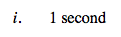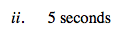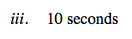### Home > CALC > Chapter Ch2 > Lesson 2.1.1 > Problem2-13

2-13.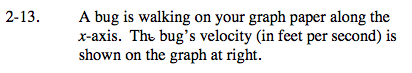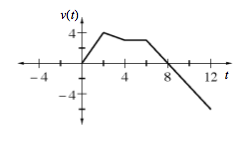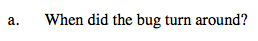Recall that positive velocity represents moving forward (away from the starting point) and negative velocity represents moving backwards (towards the starting point).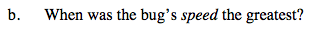Speed is the absolute value of velocity. All negative velocities become positive.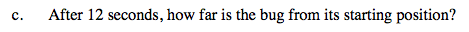Distance from the starting point (i.e. displacement) represents the accumulated distance traveled. This bug moved both forward and backwards. Be sure to consider both positive and negative travel.

Distance from the starting point (i.e. displacement) can be represent by the area under a velocity curve. Compute this area using geometry. Recall that negative area means the bug is moving backwards.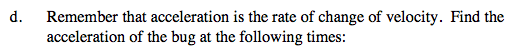$\text{rate of change of velocity }= \frac{\text{velocity}}{\text{time}}= \text{slope}$